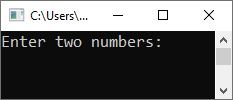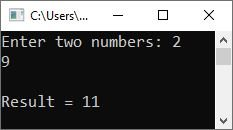# C++ Function Prototype and Definition

In this article, you will learn about the two important topics regarding the "C++ functions" which are:

Before a function can be used (called) anywhere in a C++ program, it must be declared (a function prototype) and defined (a function definition).

## C++ function prototype

A function prototype is a declaration of the function that tells the program about the type of value returned by the function and the number and type of arguments.

Function prototyping is one very useful feature of C++ functions. A function prototype describes the function interface to the compiler by giving details such as the number and type of arguments and the type of return values.

The prototype declaration looks just like a function definition, except that it has no body, i.e., its code is missing. This is the first time you knew the difference between a declaration and a definition.

A declaration introduces a function name to the program, whereas a definition is a declaration that also tells the program what the function is doing and how it is doing it.

Thus, the following are declarations, or, shall we say, function prototypes:

```int absval(int a);
int gcd(int n1, int n2);```

Therefore, you can say that a function prototype has the following parts:

• return type
• name of the function
• argument list

Let's look at the following function prototype:

`int sum(int n1, int n2);`

Here,

• return type: int
• name of the function: sum
• argument list: (int n1, int n2)

Note: Each function prototype is followed by a semicolon. A function declaration can skip the argument names, but a function definition cannot.

### C++: Use of void

The void data type specifies an empty set of values. As a result, the declaration of a function that does not return a value looks like this:

`void function_name(list of parameters);`

One can ensure that a function cannot be used in an assignment statement by declaring the function's return type to be void.

A function that does not require any parameters (i.e., it has an empty argument list) can be declared as follows:

`return_type function_name(void);`

Note: If you don't include the type specifier for a function, it is assumed that the function will return integer values. The type specifier is required to be provided for any functions that return values that are not integers.

## C++ function definition

A function must be defined before it can be invoked. The function definition contains codes that specify the function's work. That is, we define what the function will do in the program through the function definition. The general form of the function definition is as follows:

```return_type function_name(list of parameters)
{
// body of the function
}```

The "return_type" specifies the type of value that the return statement of the function returns. It may be any valid C++ data type. If no type is specified, the compiler assumes that the function returns an integer value. The "function_name" is the name of the function. The "list of arguments" is a comma-separated list of a function's variables. The "body of the function" contains the set of statements that define the work of the function. For example,

```int sum(int x, int y)
{
int res;
res = x+y;
return res;
}```

The above code snippet from the "function definition" defines the function named "sum()." And based on its definition, the function "sum()" takes two arguments and copies the values to the "x" and "y" variables, whose addition result will be initialized to the "res" variable. And finally, using the "return statement," the value of "res" will be returned to the calling function. For example:

```#include<iostream>
using namespace std;

int sum(int x, int y);          // function prototype

int main()
{
cout<<"Enter two numbers: ";
cin>>num1>>num2;

add = sum(num1, num2);       // function call

cout<<endl;

return 0;
}
int sum(int x, int y)           // function definition
{
int res;
res = x+y;
return res;
}```

The following snapshot shows the initial output produced by the above C++ program.Now input a number, say 2, and hit the ENTER key. Input another number, say 9, and hit the ENTER key again to produce the following output:Let me explain the above program—only the main section—step by step.

Step No. 1: The following statement

`int sum(int x, int y);`

Step No. 2: Declared the function with an "int" return type, the name "sum," and two "int" parameters. And the following block of code:

```int sum(int x, int y)
{
int res;
res = x+y;
return res;
}```

Step No. 3: defines the function "sum()." Now using the following code (inside the main()):

`sum(num1, num2);`

Step No. 4: The function "sum()" is called by passing the values of "num1" and "num2" as its two parameters. Therefore, the values of "num1" and "num2" are copied into the variables "x" and "y" in the function definition part. Therefore, the value of "x+y" (which equals "num1+num2") is initialized to "res," and using the "return" statement, the value of "res" will be returned to the calling function. Therefore, at the end, "res" will be initialized to "add" inside the main() function. And the value of "add" is printed on the output console.

C++ Quiz

« Previous Tutorial Next Tutorial »